Latest Teaching jobs   »   CTET 2016 Maths Pedagogy Questions: Free...

# CTET 2016 Maths Pedagogy Questions: Free PDF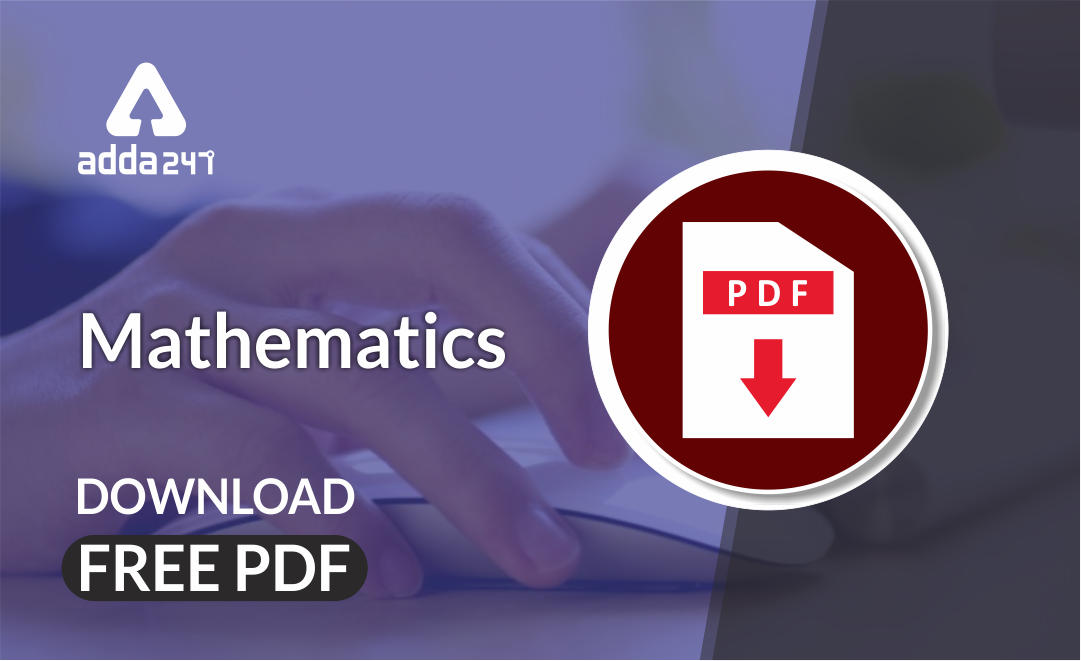Mathematics is an equally important section for CTET, MPTET, KVS & DSSSB Exams and has even more abundant importance in some other exams conducted by central or state govt.

To let you make the most of Mathematics section, we are providing important previous year Maths Pedagogy Questions.  We wish you all the best of luck to come over the fear of the Mathematics section.

Mathematics Study Notes For All Teaching Exams

## CTET 2016(Paper 2) Maths Pedagogy Questions

Q1. Anisha and Amit study in Class VII. Anisha told Amit that if the marks in Mathematics of each student in the class are increased by 5, the average would go up by 5. She further says that it is true for all numbers. Amit does not agree and Anisha proves it by taking the case n, instead of 5. Anisha is using

(a) inductive logic

(b) estimation

(c) deductive logic

(d) common sense

Q2. Using technology – based games involving factorization of numbers or formation of new shapes by joining 2D shapes, etc.

(a) enhances students’ numeracy skills and computational skills.

(b) helps teacher to manage students in Mathematics laboratory

(c) enhances students’ ability to understand the concepts better as they are able to explore,

observe and infer at their own pace.

(d) helps teacher to assess students’ performance in Mathematics.

Q3. Writing proofs in geometry implies

(a) two – column table of axioms and deductions.

(b) steps of drawing a figure.

(c) argument or justification of statements.

(d) description of a geometrical problem.

Q4. “Two complementary ‘angles are in the ratio 2 : 3. Find these angles.” The above problem from NCERT textbook of Class VII refers to

(a) lower order thinking as it is based on recalling information.

(b) higher order thinking as it demands construction of a figure using given information.

(c) lower order thinking as it is based on using the information in concrete situation.

(d) higher order thinking as it demands the interpretation of given information, its analysis and use in getting the desired information

Q5. “Prove that there is no rational number whose square is 2” This type of proof is . . . . . . . .

(a) direct proof.

(b) proof by contraposition.

(d) proof by counter-example

Q6. Consider the following statement “If the diagonals of a quadrilateral bisect each other, then the quadrilateral is a parallelogram” This statement is a / an

(a) theorem

(b) definition

(c) axiom

(d) proposition

Q7. ‘Representation’ in Mathematics does not refer to

(a) expressing the relation between two variables as an equation.

(b) expressing the number sequence through geometrical patterns.

(c) expressing an important geometrical result as a theorem.

(d) expressing the given data through graphs.

Q8. In NCERT exemplar book for Class VIII at the end of Unit 5, ‘Understanding quadrilaterals and practical geometry, lots of activities like constructing tessellation, constructing tangrams, etc., are given. One of the objectives of such tasks is to help

(a) visual learners only to improve their analytical skills.

(b) all learners with different learning styles and to enhance spatial orientation.

(c) kinesthetic learners only to improve their visual thinking skills.

(d) auditory learners only to improve their creative skills

Q9. Which one of the following statements is not true about projects in Mathematics?

(a) They promote inquiry skills.

(b) They make scoring easy in Mathematics.

(c) They enhance problem-solving skills.

Q10. Which one of the following activities is not appropriate for ‘data representation and data interpretation’?

(a) Project

(b) Newspaper report

(c) Survey

(d) Debate

Q11. Some students of your class are repeatedly not able to do well in mathematics examinations and tests. As a teacher you would:

(a) make them sit with high achievers

(b) explain the consequences of not doing well

(c) give more tests for practice

(d) diagnose the causes and take steps for remediation

Q12. In an inclusive mathematics classroom, what would be your strategy for addressing the needs of visually challenged learners?

(a) Pair them with high scorers in mathematics.

(b) Design alternate teaching-learning and assessment methods.

(c) Send the learners to a special educator

(d) Offer them another subject Rom lieu of mathematics

Q13. Which of the following assessment strategies can be used to assess learners’ interest in and attitude to mathematics?

(a) Portfolio, Project, Paper – pencil test

(b) Oral test, Paper – pencil test, Class participation

(c) Checklist, Portfolio, Paper – pencil test

(d) Checklist, Portfolio, Project, Class participation

Q14. Neeta, a Class VII mathematics teacher, assigns a lot of survey – based projects to mathematics classrooms. The purpose of such activity is:

1. to promote problem – solving skills
2. to give an opportunity to students to collect authentic data
3. to break the monotony of the classroom
4. to use it as an alternate assessment

Which of the above purposes are true?

(a) A, B and D

(b) A, B and C

(c) A and B

(d) A and C

Q15. Which of the following terms in mathematics is defined?

(a) Plane

(b) Point

(c) Line

Q16. Which of the following is not an important aspect in ‘algebra’?

(a) Generalization

(b) Visualization

(c) Measurement

(d) Transposition

Q17. ‘Problem posing’ in mathematics means:

(a) creating problems from the content

(b) inability to solve problems

(c) raising doubts in the class

(d) solving problems

Q18. A good mathematics textbook contains a lot of:

(a) theorems and proofs

(b) questions for exploration

(c) questions for practice

(d) worked out examples

Q19. Consider the following statement: “Every odd natural number is a prime number.” Which of the following methods of ‘proof’ can be used to prove / disprove the above statement?

(b) Method of disproof

(c) Direct proof

(d) Proof by contrapositive

Q20. Which of the following is not a contributing factor responsible for mathematics anxiety?

(a) Curriculum

(b) Nature of subject

(c) Gender

(d) Examination system

Mathematics Quiz For CTET Exam : Attempt Daily Quizzes

Solutions

S1. Ans.(a)

Sol. Anisha is using inductive logic.

S2. Ans.(c)

Sol. It enhances students’ ability to understand the concepts better as they are able to explore, observe and infer at their own pace.

S3. Ans.(c)

Sol. Writing proofs in geometry implies argument or justification of statements.

S4. Ans.(d)

Sol. The above problem from NCERT textbook of Class VII refers to higher order thinking as it demands the interpretation of given information, its analysis and use in getting the desired information.

S5. Ans.(c)

Sol. This type of proof is proof by contradiction.

S6. Ans.(a)

Sol. “If the diagonals of a quadrilateral bisect each other, then the quadrilateral is a parallelogram” This statement is theorem.

S7. Ans.(c)

Sol.  ‘Representation’ in Mathematics does not refer to expressing an important geometrical result as a theorem.

S8. Ans.(b)

Sol. One of the objectives of such tasks is to help all learners with different learning styles and to enhance spatial orientation.

S9. Ans.(b)

Sol. Projects in Mathematics promote inquiry skills, enhance problem-solving skills and establish interdisciplinary linkages.

S10. Ans.(d)

S11. Ans.(d)

Sol. As a teacher you would diagnose the causes and take steps for remediation.

S12. Ans.(b)

Sol. Design alternate teaching-learning and assessment methods.

S13. Ans.(d)

Sol. Checklist, Portfolio, Project, Class participation assessment strategies can be used to assess learners’ interest in and attitude to mathematics.

S14. Ans.(a)

Sol. The purpose of such activity is to promote problem – solving skills, to give an opportunity to students to collect authentic data and to use it as an alternate assessment.

S15. Ans.(d)

Sol. Diagonal of a quadrilateral in mathematics is defined.

S16. Ans.(c)

Sol. Generalization, Visualization and Transposition are important aspects in ‘algebra’.

S17. Ans.(a)

Sol. ‘Problem posing’ in mathematics means creating problems from the content.

S18. Ans.(b)

Sol. A good mathematics textbook contains a lot of questions for exploration.

S19. Ans.(b)

Sol. Method of disproof

S20. Ans.(c)

Sol. Curriculum, nature of subject and examination system are contributing factors responsible for mathematics anxiety.

•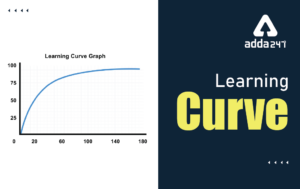Learning Curve - What is Learning Curve?...
•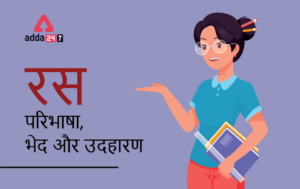रस - परिभाषा, भे�...
•पठन कौशल- परिभा�...
•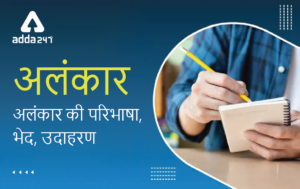अलंकार - परिभाष�...
•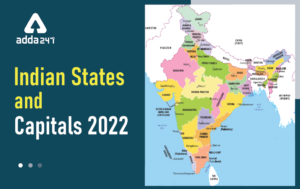Indian States and Capitals 2022: 28 Stat...
•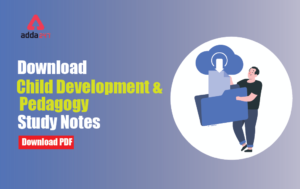New Education Policy(NEP) PDF - नई �...